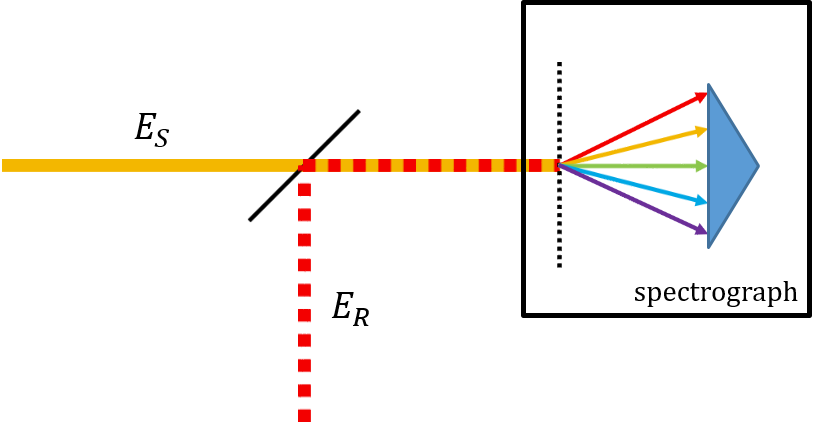# Spectral Interferometry

Capturing the phase of light

## Photons and phase

Light has properties of both particles (photons) and waves (electromagnetic fields). For time-resolved spectroscopy, the wave description is of particular interest and utility. Unfortunately, the detectors available for measuring visible light are fundamentally “photon detectors” (also known as “square-law” detectors1) since they measure the intensity ($I \propto E^2$) of light rather than its field ($E$). This is because the frequency of visible light is too high (hundreds of terahertz) for detectors to respond to the extremely fast oscillations of the electric field like an antenna does for radio waves. Intensity is basically a measure of power, which means that information about the light wave’s phase is lost (as implied by squaring the field).Schematic of spectral interferometry. A signal field ($E_S$) and reference field ($E_R$) are combined, and then spectrally resolved and detected in a spectrograph.

For many nonlinear spectroscopy techniques, we need to measure the complete electric field of the signal, including its phase. Luckily, there are methods for achieving this. One particularly powerful approach is known as spectral interferometry (SI).2 SI involves spatially overlapping a known “reference” field with the unknown signal field and then measuring the spectrum of the combined fields (known as a spectral interferogram). The resulting spectral interference encodes the magnitude and phase of the signal field, which can be extracting using the Fourier-transform spectral interferometry (FTSI) algorithm.3

## Mathematical basis of spectral interferometry

A spectral interferogram of spatially-overlapped signal $\hat{E}_s$ and reference $\hat{E}_r$ fields is measured by a spectrally-resolved square-law detector (i.e., $I \propto E^2$), yielding the intensity of the sum of the fields as a function of angular frequency ($\omega = 2\pi \nu$) $$I_{sr}(\omega) = \left| \hat{E}_s(\omega) + \hat{E}_r(\omega) \right|^2 . \tag{1}$$ This can be expanded to yield $$I_{sr}(\omega) = \left| \hat{E}_s(\omega) \right|^2 + \left| \hat{E}_r(\omega) \right|^2 + \hat{E}_s(\omega)\hat{E}_r^*(\omega) + \hat{E}_s^*(\omega)\hat{E}_r(\omega) \tag{2}$$ where the first two terms are the spectra of the signal and reference fields and the last two terms are the “interference terms”, which contain the phase information.

Notes on notation

• Variables topped with a circumflex (e.g., $\hat{z}$) are complex-valued.
• $\left| \hat{z} \right|$ is the absolute value of complex quantity $\hat{z}$.
• $\hat{z}^*$ is the complex conjugate of $\hat{z}$.
• A complex number $\hat{z}$ can be expressed in terms of its real $a = (\hat{z} + \hat{z}^*)/2$ and imaginary $b = (\hat{z} - \hat{z}^*)/2$ parts or its absolute value $\left| z \right| = (\hat{z}\hat{z}^*)^{1/ 2}$ and phase $\phi$: $$\hat{z} = a + ib = \left| \hat{z} \right| \exp (i \phi)$$

We can rewrite the first interference term as

\begin{aligned} \hat{E}_s(\omega)\hat{E}_r^* (\omega) & = \left| \hat{E}_s(\omega) \right| \exp \left[ i \phi_s(\omega) \right] \times \left\{ \left| \hat{E}_r(\omega) \right| \exp \left[ i \phi_r(\omega) \right] \right\}^* \\ & = \left| \hat{E}_s(\omega) \hat{E}_r(\omega) \right| \exp \left[ i \left\{ \phi_s(\omega) - \phi_r(\omega) \right\} \right] \\ & = \left| \hat{E}_s(\omega) \hat{E}_r(\omega) \right| \exp \left[ i \Delta\phi(\omega)\right] \end{aligned} \tag{3}

where $\Delta\phi(\omega) = \phi_s(\omega) - \phi_r(\omega)$ is the relative phase of the signal and reference fields.4 We can express $\Delta\phi$ in terms of a linear phase (i.e., delay) and the sum of all other components:

$$\Delta\phi(\omega) = \omega (t_s - t_r) + \Delta\phi^\prime(\omega) . \tag{4}$$

Using this new form for $\Delta\phi$, the interference term becomes

$$\hat{E}_s(\omega)\hat{E}_r^*(\omega) = \left| \hat{E}_s(\omega) \hat{E}_r(\omega) \right| \exp \left[ i \omega (t_s - t_r) \right] \exp \left[ i \Delta\phi^\prime(\omega)\right] . \tag{5}$$

Therefore, by the Fourier modulation theorem5, when the reference field is delayed relative to the signal field (i.e., $t_s - t_r < 0$), the interference term $\hat{E}_s(\omega)\hat{E}_r^*(\omega)$ appears as a peak on the negative side of $t = 0$ once Fourier transformed to the time domain.

## Fourier-transform spectral interferometry algorithm

Let’s simulate a spectral interferogram between an exponentially-damped sinusoidal “signal” field $E_s(t)$ and a “reference” field with a Gaussian temporal field envelope $E_r(t)$. The signal field is given by

$$E_s(t) =\mathrm{Re}\left\lbrace \mathrm{exp}\left\lbrack \left(2\pi if_s^0 -\frac{1}{\tau_s }\right)t\right\rbrack H\left(t\right)\right\rbrace$$

where $\mathrm{Re}\lbrace\hat{z}\rbrace$ selects the real part of complex value $\hat{z}$, $f_s^0$ is the signal center frequency, $\tau_s$ is the exponential damping time constant, $t$ is time, and $H(t)$ is the Heaviside step function.6 The reference field is given by

$$E_r(t) =\mathrm{Re}\left\lbrace G\left(t;T_r ,\mathrm{FWHM}\right)\;\mathrm{exp}\left\lbrack 2\pi if_r^0 \left(t-T_r \right)\right\rbrack \right\rbrace$$

where $G(t; T_r, \mathrm{FWHM})$ is a Gaussian function of $t$ with delay $T_r$ and full-width at half-maximum $\mathrm{FWHM}$, and $f_r^0$ is the reference center frequency. These fields are plotted below.

For the sake of example, we will use the following parameter values:

$f_s^0$$0.375 \,\mathrm{fs}^{-1} \ (375 \,\mathrm{THz}) \tau_s$$30 \,\mathrm{fs}$
$f_r^0$$0.375 \,\mathrm{fs}^{-1} \ (375 \,\mathrm{THz}) \mathrm{FWHM}$$15 \,\mathrm{fs}$